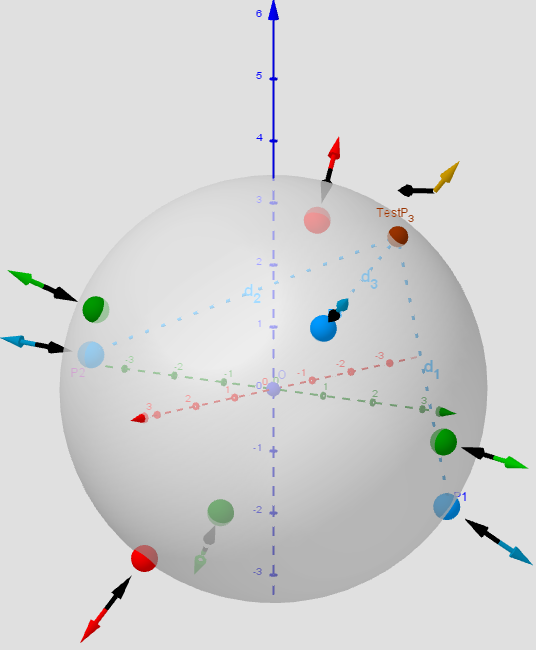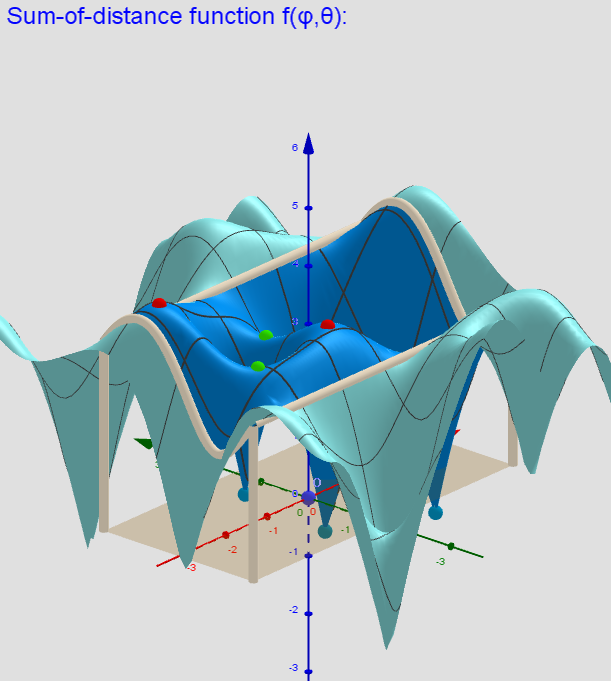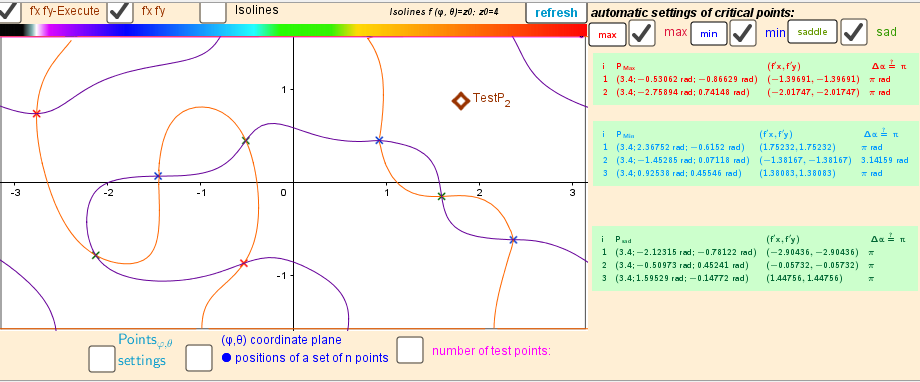# Images. Finding Geometric Medians on a bounded area

Let Pi=(xi,yi,zi) n moving points in ℝ³ (lP:={P1,P2,...,Pn}). I want to find the points P=(x,y,z) on the surface of the sphere -S (radius R) that are critical (relative min/max or saddle points at (x,y,z)) of a function f(x,y,z):=-sum of the distances from P to the all points from lP. Critical points can be found using Lagrange multipliersas finding the Extreme values of the function f(x,y,z) subject to a constraining equation g(x,y,z):=x2+y2+z2-R2=0. There is a system of equations: ∇f(x,y,z)= λ∇g(x,y,z). A local optimum occurs when ∇f(x,y,z) and ∇g(x,y,z) are parallel, and so ∇f is some multiple of ∇g. This applet illustrates distributions of geometric medians on a sphere of radius R "induced" by a discrete sample of moving points in three-dimensional space. Description in https://www.geogebra.org/m/y8dnkeuu Applets: Example of Applet in which 3 moving points in three-dimensional space "induce" 8 geometric medians on a sphere. Example of Applet in which 6 moving points in three-dimensional space "induce" 14 geometric medians on a sphere.Distribution of points Pi, test Point, Max/min/saddle -Critical points on a sphere. Vectors ∇f and ∇g at these points. n=3.Two-variable function f(φ,θ) over a rectangular region: - π ≤φ ≤ π; -π/2≤θ≤π/2. n=3.Intersection points of implicit functions -solutions of the Lagrange equation. n=3